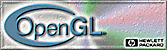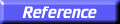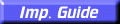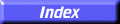# glBegin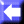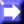glBegin, glEnd: delimit the vertices of a primitive or a group of like primitives.

##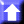C Specification

```void glBegin(
GLenum	 mode)

void glEnd(void)
```

##Parameters

mode
Specifies the primitive or primitives that will be created from vertices presented between glBegin and the subsequent glEnd. Ten symbolic constants are accepted: GL_POINTS, GL_LINES, GL_LINE_STRIP, GL_LINE_LOOP, GL_TRIANGLES, GL_TRIANGLE_STRIP, GL_TRIANGLE_FAN, GL_QUADS, GL_QUAD_STRIP, and GL_POLYGON.

##Description

glBegin and glEnd delimit the vertices that define a primitive or a group of like primitives. glBegin accepts a single argument that specifies in which of ten ways the vertices are interpreted. Taking n as an integer count starting at one, and n as the total number of vertices specified, the interpretations are as follows:
GL_POINTS
Treats each vertex as a single point. Vertex n defines point n. n points are drawn.
GL_LINES
Treats each pair of vertices as an independent line segment. Vertices 2n
1 and 2n define line n. n/2 lines are drawn.
GL_LINE_STRIP
Draws a connected group of line segments from the first vertex to the last. n1 lines are drawn.
GL_LINE_LOOP
Draws a connected group of line segments from the first vertex to the last, then back to the first. Vertices n and n+1 define line n. The last line, however, is defined by vertices n and 1. n lines are drawn.
GL_TRIANGLES
Treats each triplet of vertices as an independent triangle. Vertices 3n2, 3n1, and 3n define triangle n. n/3 triangles are drawn.
GL_TRIANGLE_STRIP
Draws a connected group of triangles. One triangle is defined for each vertex presented after the first two vertices. For odd n, vertices n, n+1, and n+2 define triangle n. For even n, vertices n+1, n, and n+2 define triangle n. n2 triangles are drawn.
GL_TRIANGLE_FAN
Draws a connected group of triangles. One triangle is defined for each vertex presented after the first two vertices. Vertices 1, n+1, and n+2 define triangle n. n2 triangles are drawn.
Treats each group of four vertices as an independent quadrilateral. Vertices 4n3, 4n2, 4n1, and 4n define quadrilateral n. n/4 quadrilaterals are drawn.
Draws a connected group of quadrilaterals. One quadrilateral is defined for each pair of vertices presented after the first pair. Vertices 2n1, 2n, 2n+2, and 2n+1 define quadrilateral n. n/21 quadrilaterals are drawn. Note that the order in which vertices are used to construct a quadrilateral from strip data is different from that used with independent data.
GL_POLYGON
Draws a single, convex polygon. Vertices 1 through n define this polygon.
Only a subset of GL commands can be used between glBegin and glEnd. The commands are glVertex, glColor, glIndex, glNormal, glTexCoord, glEvalCoord, glEvalPoint, glArrayElement, glMaterial, and glEdgeFlag. Also, it is acceptable to use glCallList or glCallLists to execute display lists that include only the preceding commands. If any other GL command is executed between glBegin and glEnd, the error flag is set and the command is ignored.

Regardless of the value chosen for mode, there is no limit to the number of vertices that can be defined between glBegin and glEnd. Lines, triangles, quadrilaterals, and polygons that are incompletely specified are not drawn. Incomplete specification results when either too few vertices are provided to specify even a single primitive or when an incorrect multiple of vertices is specified. The incomplete primitive is ignored; the rest are drawn.

The minimum specification of vertices for each primitive is as follows: 1 for a point, 2 for a line, 3 for a triangle, 4 for a quadrilateral, and 3 for a polygon. Modes that require a certain multiple of vertices are GL_LINES (2), GL_TRIANGLES (3), GL_QUADS (4), and GL_QUAD_STRIP (2).

glArrayElement,
glCallList,
glCallLists,
glColor,
glEdgeFlag,
glEvalCoord,
glEvalPoint,
glIndex,
glMaterial,
glNormal,
glTexCoord,
glVertex